# The History of Weather Forecasting

CONTENTS
 1 Introduction 2 Early Forecasting 2.1 First Attempts 2.2 Establishing Weather Services 3 Numerical Weather Prediction 3.1 Finding the Maths 3.2 Computers and Chaos 3.3 Primitive Equations 4 Forecasting Models Used Today 4.1 The Finite Difference Method 4.2 The Spectral Method 4.3 The Finite Element Method 4.4 Current Research 5 Conclusion
1)      Introduction

It is one of the most common topics of conversation, it has influenced people's lives for thousands of years, and predicting it requires the most powerful computers on the planet: the weather. Most ancient cultures include weather gods, and weather catastrophes have an important role in creation myths of many cultures, for example the Deluge described in the Bible . Observing the skies and drawing the correct conclusions from these observations was crucial to people's survival . Nowadays, we are more independent of weather conditions due to central heating, air conditioners, greenhouses and so forth, but weather forecasts are more accurate than they ever were . Forecasts, both for the next couple of hours and for the next couple of days, are issued daily. Apart from helping people decide when they should invite their neighbours for a barbecue, weather forecasts provide vital information for a wide range of occupational categories such as farmers, pilots, sailors and soldiers. Furthermore, thanks to forecasts, people are less likely to be surprised by severe weather; and people suffering from hay fever for example can time their outdoor activities according to pollen flight forecasts. The great success of the weather channel in U.S. television and the fact that weather reports often have a higher audience rate than the preceding news broadcasts, illustrate that forecasting has become a highly competitive business .
But how are weather forecasts created? And why has forecast accuracy improved so dramatically over the last couple of decades? In this article, I will examine the history of weather forecasting, and especially the historical development of the use of mathematics in forecasting, before describing the basic principles of the mathematical methods used in current forecasting models. I will also present some of the current research projects in weather forecasting.

Chapter 2 of the article provides an overview of early forecasting attempts, from the first observations made by ancient cultures to the development of government-supported weather services.

Chapter 3 reviews the use of mathematics in weather forecasting, starting with the fathers of numerical weather prediction, Vilhelm Bjerknes and Lewis Fry Richardson. Then, the seminal work of Carl-Gustaf Rossby is treated briefly, before the use of computers in weather forecasting, pioneered by Jule Charney (and others), and the application of chaos theory to meteorology by Edward Lorenz are discussed. Moreover, the primitive equations describing atmospheric processes, which are used in forecasting models, are presented and explained as well.

Chapter 4 presents the two most important mathematical methods used in forecasting today, namely the finite difference method and the spectral method, along with the corresponding grid models applied to the atmosphere. The finite element method, which is another method for numerically solving partial differential equations, is described briefly. An overview of some of the current research projects and research areas in weather forecasting is also given.

This article should be understood by undergraduate maths or physics students who have taken 2000-level maths courses offered by the university. The reader does not need to have much background in applied mathematics or physics, but is expected to know basic physical principles, for instance gravity, centrifugal force, friction and waves. Furthermore, I have assumed that the reader is familiar with differential equations, differentiation of functions of several variables, Fourier series and Gaussian elimination. Explaining these concepts would have gone beyond the scope of this article. For similar reasons I have chosen not to explain less familiar methods, for instance the Galerkin, the least-square and the Runge-Kutta methods. The reader is kindly asked to look up these methods (and any other unfamiliar mathematical concepts) in appropriate textbooks. However, as the article should provide only an overview of the mathematical methods used in current forecasting models, I have chosen to include only simple equations and explain some mathematical symbols in order to make understanding the methods easier. Also, a number of mathematical concepts are explained in words rather than with equations. While I have assumed that the reader has a good grounding in general knowledge, I explain several meteorological terms.

The aim of the article is to give the reader a summary of the historical development of both weather forecasting in general as well as the application and the importance of mathematics in forecasting in particular. In addition, it should give an impression of how sophisticated mathematical models have to be in order to accurately simulate and predict the state of the atmosphere and hence the weather.

2)      Early Forecasting

Weather has always played an important role in people's lives, and attempts to predict weather changes were made early on. For a very long time, forecasts were based on observations of the skies both during the day and at night. From the 17th century onwards, scientists were able to measure factors related to weather such as pressure and temperature. This helped them understand the atmosphere and its processes better, and they began to collect weather observation data systematically. By the end of the nineteenth century, several European countries and the United States had established the first weather services.

2.1)      First Attempts

It is not known when people first started to observe the skies, but at around 650 BC, the Babylonians produced the first short-range weather forecasts, based on their observations of the stars and clouds. The Chinese also recognised weather patterns, and by 300 BC astronomers had developed a calendar which divided the year into 24 festivals, each associated with a different weather phenomenon. Generally, weather was attributed to the vagaries of the gods, as the wide range of weather gods in various cultures, for example the Egyptian sun god Ra and Thor, the Norse god of thunder and lightning, proves. Many ancient civilisations developed rites such as rain dances and animal sacrifices in order to propitiate the weather gods.

The ancient Greeks were the first to develop a more scientific approach to explaining the weather. The work of the philosopher and scientist Aristotle (384-322 BC) is especially noteworthy, as it dominated people's views on and their knowledge of the weather for the next 2000 years. In 340 BC, Aristotle wrote his book Meteorologica , where he tried to explain the formation of rain, clouds, wind and storms. In addition, he also described celestial phenomena such as comets and haloes. Many of his observations were -- in retrospect -- surprisingly accurate. For example, he believed that heat could cause water to evaporate . But he also jumped to quite a few wrong conclusions, such as that winds form "as the Earth exhales" , which were rectified from the Renaissance onwards.

Throughout the Middle Ages and beyond, the Church was the only official institution that was allowed to explain the causes of weather, and Aristotle's Meteorologica was established as Christian dogma [1, p. 7]. Besides, weather observations were passed on in the form of rhymes, which are now known as weather lore. Many of these proverbs are based on very good observations and are accurate, as contemporary meteorologists have discovered .

By the end of the Renaissance, scientists realised that it would be much easier to observe weather changes if they had instruments to measure fundamental quantities in the atmosphere such as temperature, pressure and moisture. Until then, the only tools available were vanes, to determine the wind direction, and early versions of rain gauges. In 1592, Galileo Galilei (1564-1642) developed the world's first thermometer. His student Evangelista Torricelli (1608-1647) invented the barometer in 1643, which allowed people to measure atmospheric pressure. Five years later, Blaise Pascal (1623-1662) proved that pressure decreases with altitude. This discovery was verified by Edmond Halley (1656-1742) in 1686; Halley also was the first one to map trade winds. The discoveries of unknown continents and, by extension, of unknown climates and weather phenomena also played a part in the development of modern meteorology .

Gradually, scientists began to understand that weather is influenced by large-scale atmospheric processes and that wind and storms for example follow certain patterns. One of the first to study storms was the scientist and politician Benjamin Franklin (1706-1790). His most famous scientific achievement is his work on electricity and lightning in particular, but in fact he was very interested in weather and studied it throughout most of his life. For example, Franklin discovered that storms generally travel from west to east. Many of Franklin's observations paved the way to a better understanding of climate and the atmosphere, but
... Franklin was still a natural philosopher at heart, and he was not inclined to clutter his conjectures with a lot of data or mathematics [1, p. 10].
Predicting the weather was still pigeonholed as a pipe dream and any serious scientist would engage in studying weather phenomena only in their spare time, if at all. All conjectures and theories on weather were based solely on observations and it was not until the early 20th century that mathematics and physics became part of meteorology. However, by the mid-eighteenth century, governments in various European countries and in the United States realised that accurate weather forecasts can considerably help to save money and even lives. Consequently, the first weather services were established.

2.2)      Establishing Weather Services

One of the first weather observation networks, which operated from 1654 to 1670, was established by the Tuscan nobleman Ferdinand II . More than 100 years later, the Palatine meteorological society (Societas Meteorologica Palatina) installed a first global weather archive. For fifteen years (1780-1795), 39 weather stations, spread across Northern America, Europe and Russia, collected meteorological data three times per day, using standardised equipment . The society did not produce any forecasts; one of the main obstacles for this was that the data had to be sent to the society's main office by boat and post coaches, which took weeks.

The invention of the electrical telegraph in 1837 by Samuel Morse facilitated the production of weather forecasts, as data and any other weather observations now could easily and swiftly be transmitted to another country and even to another continent. Observation wards began to appear all over Europe and Northern America, but it was not until the Crimean War (1853-1856) that people realised the benefits of weather forecasts. The fleet of the Ottoman Empire was surprised by a low-pressure system and, as a result, lost several ships. The French Emperor Napoleon III later ordered that the weather for that day should be analysed; he learned that the storm could have been predicted and that warnings could have been transmitted by telegraph . Hence, the loss of the ships could have been prevented.

Meteorologists had begun to map data from various observation stations, such as temperature and humidity, onto weather charts (see figure 1). These weather maps enabled the scientists to detect and study storm systems and wind patterns as well as comparing the current meteorological situation to past ones, which ultimately led to the production of forecasts. This method, based on the analysis and comparison of many standardised observations taken simultaneously, is called synoptic weather forecasting.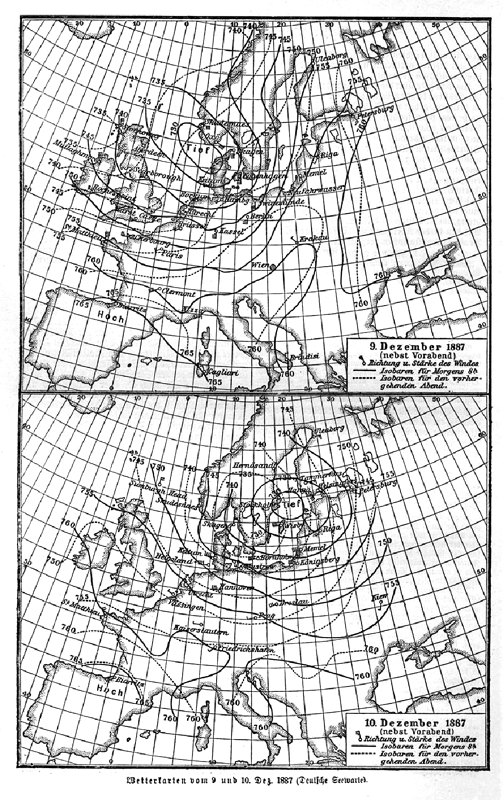Weather maps for Europe, 09. and 10. December 1887
Click on the picture to see a larger version

It is still used today by weather services both as a starting point for and as an addition to numerical models. Several European governments and later also the US government realised that timely storm warnings could prevent ship losses. Consequently, storm-warning systems were installed. The British Meteorological Department issued regular gale warnings from 1861 onwards; the first US storm-warning system began to operate ten years later, dwarfing the European services with its size and funds . These institutions went on to produce general weather forecasts.

In the 1920s, weather maps became much more detailed due to the invention of the radiosonde: a small lightweight box containing measurement equipment and a radio transmitter. It is attached to a hydrogen-filled weather balloon that can ascend to up to 30 km before bursting. The radiosonde transmits humidity, pressure, temperature, and wind speed measurements to a ground station. Of course, radiosondes and weather balloons have been improved substantially over the last 80 years, but even the early models allowed meteorologists to observe weather conditions in high altitudes.
Modern weather services depend on observations transmitted both by radiosondes and by satellites. The first weather satellite, TIROS 1 (Television and Infrared Observation Satellite) was launched in 1960. Satellites collect data, similarly to radiosondes, but they have the great advantage that they can cover areas difficult to access such as oceans and deserts. Today, both polar orbiting satellites (at an altitude of 800-900 km; they revolve around the Earth following the degrees of longitude, passing over both poles) and geostationary satellites (at an altitude of 35,800 km  directly above the equator; they travel in time with the Earth's rotation, thus constantly observing the same area) are used.
Still, what improved weather forecasting much more than new observation tools, was implementing a new forecasting method called numerical weather prediction.

3)      Numerical Weather Prediction

Both the financial significance of weather forecasts and the necessity of knowing more about atmospheric processes were understood fairly soon after the first gale warnings were published, but very few people realised that mathematics could be used to describe these processes and produce more accurate forecasts than synoptic meteorology ever could. In the early 20th century, scientists, in particular Vilhelm Bjerknes and Lewis Fry Richardson, pioneered numerical weather forecasting, which is based on applying physical laws to the atmosphere and solving mathematical equations associated to these laws. The discovery of chaos theory and not least the development of computers greatly improved the quality of forecasts. Today, meteorologists constantly refine the various forecasting models designed by the world's leading weather services.

3.1)      Finding the Maths

The first mathematician who thought of applying mathematics to weather forecasting was the Norwegian Vilhelm Bjerknes (1862-1951). Already at a young age, Bjerknes engaged in mathematics as he assisted his father with his research in hydrodynamics. He then studied mathematics and physics at the University of Christiania (nowadays Oslo). In 1898 he formulated his circulation theorem: in a nutshell, it explains the evolution and the subsequent decay of circulations in fluids. Possibly even more importantly, the theorem also marks "the move of Vilhelm Bjerknes into meteorology" [11, p. 479]. Combining his circulation theorem with hydrodynamics and thermodynamics, Bjerknes discovered that, given initial atmospheric conditions, it is possible to compute the future state of the atmosphere using mathematical formulae. As he put it himself [1, p. 152]:
We must apply the equations of theoretical physics not to ideal cases only, but to the actual existing atmospheric conditions as they are revealed by modern observations. ...From [these] conditions ... we must learn to compute those that will follow.
During a visit to the United States in 1905, he presented his theories. The Carnegie Foundation was so impressed by his ideas that it funded Bjerknes's research for the next 36 years. Bjerknes founded the Bergen Geophysical Institute, also known as the Bergen School, which would make several important contributions to modern meteorology. Together with his son Jacob, who became a famous meteorologist himself, and meteorologists Tor Bergeron and Halvor Solberg, he discovered that weather patterns are closely associated with so-called fronts, i.e. transition zones between warm and cold air masses, and described the life cycle of mid-latitude cyclones. Most of Bjerknes's important results were published in On the Dynamics of the Circular Vortex with Applications to the Atmosphere and to Atmospheric Vortex Wave Motion in 1921.

Bjerknes' equations were very complicated and not very practical for predicting the weather as they required immense computational power, a fact of which he was aware himself. Nevertheless, he firmly believed that one day, meteorology would be a proper science and weather forecasts based on solving mathematical equations would be feasible:
It could take years to drill a tunnel through a mountain. Many a worker will not live to witness the breakthrough. Nonetheless, this will not bar others from later riding through the tunnel at express train speed. 
The first attempt to use mathematics in order to predict the weather was made by the British mathematician Lewis Fry Richardson (1881-1953), who simplified Bjerknes' equations so that solving them became more feasible. Richardson, who had studied a number of sciences at both Newcastle University and the University of Cambridge, worked in the British Meteorological Office from 1913 until 1916. In 1919, he resumed his former job there, but resigned only a year later when the Meteorological Office became part of the Air Ministry. Richardson would have needed to join the army, which was incompatible with his pacifist Quaker conviction.

He also worked for the National Peat Industries for some time, and in order to solve differential equations modelling the flow of water in peat, he invented his method for finite differences, which produces highly accurate results. Basically, this method allows finding approximate solutions to differential equations. A differential equation with a smooth variable is converted into a function (or an approximation thereof) that relates the changes of the variable and given steps in time and/or space, meaning that the changes are calculated at discrete points rather than at infinitely many points. Then the derivatives in the differential equation are replaced by finite difference approximations (this method will be explained in more detail in section 4.1). So in the place of the differential equation you get many equations which can be solved using arithmetic.

Finite difference methods are widely used nowadays, but in Richardson's days, other mathematicians considered the method to be "approximate mathematics" [8, p. xvi]. Nevertheless, when Richardson came across problems of the dynamics of the atmosphere at his work at the Meteorological Office, he decided to solve them using his method. He remodelled the fundamental equations describing atmospheric processes such that it was possible to solve them numerically.

By dividing the surface of the Earth into thousands of grid squares, and the atmosphere into several horizontal layers, he obtained a large number of grid boxes, connected to one another by mathematical equations. Fundamentally, Richardson applied Bjerknes' vision of calculating the future state of the atmosphere using observations of its current state to the grid and added to it the idea that there is a connection between the grid boxes.

Richardson imagined a "forecast factory" where thousands of human computers would be seated on galleries along the walls of a huge hall. Each computer would be responsible for calculating the changes in the atmosphere for discrete points in one grid box. In the centre of the room, there was to be a pulpit with a "conductor" who would make sure that the computers all worked at uniform speed, in time with each other. The calculations would be collected as soon as they were finished in order to be transmitted to a radio station. Richardson also included a research department in charge of refining the models [10; 1, pp. 157-158].

This forecast factory is "remarkably similar to descriptions of modern multiple-processor supercomputers used in weather forecasting today" . In Richardson's days, however, all the computations had to be done manually, and he estimated that his factory would need 64,000 human computers to master the mammoth task of calculating the weather in time with the weather actually happening. But he believed that:
Perhaps some day in the dim future it will be possible to advance the computations faster than the weather advances and at a cost less than the saving to mankind due to the information gained. But that is a dream. [1, p. 161]
In 1916, Richardson decided to join the Friends Ambulance Unit (that had been formed for conscientious objectors) and serve in the First World War, out of curiosity. He took his research with him, and when he was not transporting wounded to military hospitals, he tested his model. For this he used data collected on 20th May 1910, which had been an international balloon day where European observatories had taken measurements in the upper air, and that were provided by Vilhelm Bjerknes. It took Richardson weeks to produce a six-hour forecast, and when he compared his results to the actual measurements, he discovered that his results were not only highly inaccurate, but also absolutely unrealistic. Nonetheless, he decided to include his calculations in his seminal book Weather Prediction by Numerical Process, which was published in 1922. For him, this first failed attempt to put his model into practice was only a minor detail compared to the fact that he had developed his method in the first place. [1, p. 160]

Very few understood the importance and the novelty of Richardson's work at the time, as, as he wrote himself, "the scheme is complicated because the atmosphere is complicated" [1, p. 161]. This lack of recognition and the affiliation of the Meteorological Office to the Air Ministry led Richardson to abandon meteorology. He spent a considerable amount of the rest of his life studying the mathematics of war.

Richardson's theories were not put into practice again until the mid-1940s, when a team of scientists at the Institute for Advanced Study in Princeton developed the world's first computers. Meanwhile, the groundbreaking research of meteorologists such as Jacob Bjerknes (1897-1975) and Carl-Gustaf Rossby (1898-1957) furthered scientists' knowledge of the atmosphere and helped pave the way for the eventual triumph of numerical weather forecasting.

Rossby in particular should be credited with this, as he almost single-handedly changed the practices of the U.S. Weather Bureau, introducing forecasting methods based on meteorological and physical theories. Before moving to the United States, Rossby had worked at the Bergen Geophysical Institute under Vilhelm Bjerknes. He brought the school of thought of the Bergen scientists across the pond, where he established several meteorological centres. Apart from training young meteorologists, Rossby produced stunning research results. In 1939, when he held a professorship at the Massachusetts Institute of Technology (MIT), he discovered the so-called Rossby waves, which are meanders of large-scale airflows in the atmosphere. They form when an airflow, for example the jet stream, is deflected either to the north or to the south (by a mountain range for example), but because of the conservation of "potential vorticity", the flow returns to its original latitude. Rossby waves are very long, with only three to six oscillations around the entire planet; they play a very important role in the formation of cyclones (i.e. lows) and anticyclones (i.e. highs) [2, p. 216].

The wave speed $c$ relative to the ground is given by:where $U$ is the mean westerly wind, ω is the angular velocity of the Earth, φ is the latitude, $R_{E}$ is the radius of the Earth and λ is the wavelength [46; 4, p. 237]. The middle term on the right-hand side is the so-called Rossby parameter, which describes the variations of the Coriolis parameter with latitude, where the Coriolis parameter $f_{C}$ is given by:
$f_{C} = 2 \times \omega \times \sin \phi$
Calculating the wave speed gives the movement of the wave crests from west to east [4, p. 237]. In terms of numerical weather prediction, this equation is important as it facilitated extended five-day forecasts [1, p. 186].

The work of Vilhelm Bjerknes and Richardson had divided the meteorological world into two camps: one camp defended the old method of comparing the current state of the atmosphere with past observations; the other camp campaigned for the use of physics in weather forecasting.

A turning point was the 6th of June 1944: D-Day. For the invasion of Normandy to be a success, the commanders of the Allied forces wanted certain weather conditions (clear enough skies, relatively calm winds). Their requirements for the tide and the moon phase restrained the time span in which to undertake the invasion to early June; the exact day should be determined on the basis of weather forecasts. The commanders had employed advocators of the two opposing schools of thought in weather forecasting: the Norwegian meteorologist Sverre Petterssen, a disciple of the Bergen School, and the American Irving Krick, whose reputation as a forecaster mainly stemmed from his talent for selling himself. Throughout May 1944, the weather had been calm, and Krick, having studied old weather maps, predicted that the conditions would not change. Meanwhile, Petterssen saw heavy storms coming in from the Atlantic. The only day with conditions good enough for the invasion was 6th June [10; 1, pp. 189-195]. The commanders decided to trust Petterssen's forecast, thereby demonstrating a great deal of trust in science [1, pp. 195-196].

D-Day not only changed world history, it also highlighted the importance of weather forecasts . Soon after the Second World War, American scientists used the world's first computers to predict the weather. Other groundbreaking research results, be it the formulation of chaos theory, be it the invention of computers, significantly changed weather forecasting. Thus, numerical weather prediction began to conquer the world.

3.2)      Chaos and Computers

The mathematician John von Neumann (1903-1957), one of the fathers of computer science, was the first one to think of using computers to predict the weather. His major motive was not meteorology, though, as he thought that by predicting the weather, one might be able to control it and use it as a weapon. In 1946, he presented his ideas to a group of leading meteorologists, including Carl-Gustaf Rossby and the young, gifted mathematician Jule Charney (1917-1981).

Charney became one of the leading scientists in von Neumann's Meteorology Project at the Institute for Advanced Study in Princeton. The team developed their own computer, but ended up using the ENIAC (Electronic Numerical Integrator and Computer), which belonged to the U.S. Army, for their first forecast because their computer was not yet ready. In 1950, the ENIAC successfully produced a 24-hour forecast. This took about 24 hours, but the computer developed by von Neumann's team was much faster (this computer produced its first forecast in 1952), and from 1955 onwards, numerical forecasts generated by computers were issued on a regular basis. At first, experienced human forecasters were sceptical about the quality of these forecasts, which admittedly were not as good as forecasts made by humans, but the rapid development of computers and hence their speed dramatically improved forecast quality.

The prerequisite for computer-generated forecasts was to simplify the full primitive equations that govern the atmosphere (they will be discussed in section 3.3), as the early computers were unable to deal with all the equations included in Richardson's model. In 1948, Charney developed the quasi-geostrophic approximation, which reduces several equations of atmospheric motions to only two equations in two unknown variables [14, chapter 13]. These equations are much easier to solve and could be handled by the early computers. (Geostrophic winds are hypothetical winds for which the balance between the pressure-gradient force and the Coriolis effect is exact (see section 3.3) [2, p. 112]. In Charney's approximation, winds are assumed to be almost geostrophic.)

Furthermore, this approximation filters out all but the slow long-wave motions that are important in meteorology, so that you do not have to solve the primitive equations for acoustic and gravity waves as Richardson did 30 years earlier. Although the computers were fed with simplified equations only, the limited computer power demanded a barotropic (i.e. single-layer) model of the atmosphere. Further research, both in meteorology and in computer science, finally allowed the application of baroclinic (i.e. multi-layer) models. In 1963, a six-layer model based on the primitive equations was used for producing a forecast. Since then, as computer power increased, the models have constantly been refined (meaning that more layers, a finer grid, more equations, topography and landscape characteristics were included) [4, p. 314]. This dramatically increased both the forecast accuracy and quality.

Today, the world's leading meteorological centres use the most powerful computers on the planet; the new computer at the British Met Office for example is capable of 125 trillion calculations per second .

Meteorologists were thrilled by the possibilities that seemed to open up due the use of computers in weather forecasting, and were convinced that it would only be a matter of time before man could not only accurately predict, but also control the weather. The discovery that the atmosphere is chaotic, by MIT researcher Edward Lorenz (1917-2008) in 1961, came as a serious blow to all these high-flying ideas. As opposed to other meteorologists at the time, Lorenz did not predict the weather, but investigated how predictable it is, in other words, if there are periodic patterns (the existence of patterns would have supported the belief of some old-school meteorologists that weather forecasting based on the study of past weather events yields accurate results). For this he ran a shortened forecasting model on his computer, and to his great surprise, inputting data that differed from previously entered values only in the fourth decimal place, significantly changed the weather the computer predicted . Lorenz reasoned that the dynamical equations that describe the atmosphere are exceedingly sensitive to initial conditions. Dynamical equations are deterministic; meaning that given initial conditions, they determine how the process they describe will evolve in the future. But, in the case of the atmosphere (and many other systems as well, chaotic behaviour can be found in every branch of science), you need to enter the exact same data as initial conditions in order to get the same results if you run the model several times. Even seemingly minuscule differences in the initial conditions result in highly different outcomes. A very simplified example for chaotic behaviour is the trajectory of a paper aeroplane: Imagine you throw a paper aeroplane in a similar manner and in the same direction say ten times. Every time, the trajectory of the plane will be different, because you will never be able to throw the plane in the exact same manner twice: the force you exert or the way you hold the plane in your hand when throwing it will differ ever so slightly from throw to throw, resulting in very different, unpredictable flights.

Chaotic behaviour is commonly known as the "butterfly effect", a term coined due to the title of a talk Lorenz gave in 1972: Predictability: Does the Flap of a Butterfly's Wings in Brazil Set Off a Tornado in Texas? Meteorologists had presumed that small weather changes in some specified places would affect the weather in other places, but after Lorenz's discovery, they had to accept that it did not matter if a butterfly flapped its wings in Brazil, or Bulgaria, or Bangladesh, the result might still be a tornado in Texas (or somewhere else for that matter). This, combined with the fact that observations of the atmosphere are usually slightly erroneous, meant that long-range forecasts would not be possible, as the small errors would build up very quickly and change the outcome considerably. Lorenz believed that weather could not be forecast accurately for more than about two weeks. The current models do not even exhaust this limit, as:
After about ten days it is essentially impossible to forecast weather with any degree of accuracy. 
Modern forecast models allow for the chaotic nature of the atmosphere by a process called ensemble forecasting, meaning that the model is run several times, each time with slightly different initial conditions. The results are then averaged out to give a forecast. This method can also suggest unlikely weather conditions with quite a high degree of accuracy [4, p. 330].

Finding a balance between detailed, accurate long-range forecasts and chaotic behaviour is still a part of modern meteorological research. But before looking at current forecasting models, let us look at the primitive equations that form the basis of every such model.

3.3)      Primitive Equations

For each weather forecast, meteorologists need to know the values of seven physical quantities: temperature, pressure, density, humidity, and wind velocity, containing three components accounting for the three different wind directions. Some forecasting models also include the content of water and ice in clouds and at the ground. Ideally, these values would be known at every point in the atmosphere at any time. This is virtually impossible due to limited computational power, therefore the atmosphere is divided into many small air parcels, and the values are calculated for each parcel. Scientists treat the Earth's atmosphere as if it were a fluid on a rotating sphere in order to describe large-scale atmospheric processes using the fundamental laws of thermodynamics and hydrodynamics, also called the primitive equations. Essentially, they are the equations of motion, one for each of the three wind directions, the continuity equation, describing the conservation of mass, the ideal gas law, and the first law of thermodynamics, describing the conservation of energy. There is also an equation for determining the humidity, which is not always included in the set of the primitive equations (and is not treated here). Furthermore, the following forces have to be taken into account: gravitation, drag force, pressure gradient force, and, since the Earth is rotating, the Coriolis and the centrifugal forces [15, p. 118]. Here, only the very basic versions of the primitive equations are described.

The equations of motion are based on Newton's second law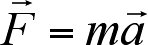: force equals the product of mass and acceleration. Basically, they describe how an object moves under different circumstances. The movement of an air parcel, i.e. the winds in a parcel, is predicted by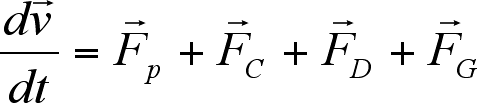whereindicates the change of velocity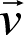with respect to the time $t$. The terms on the right-hand side represent the forces acting in the atmosphere, namely the pressure gradient force, the Coriolis force, the drag force and the gravitational force.

The pressure gradient force (per unit mass)is perpendicular to the isobars (lines on weather charts joining points where the atmospheric pressure is the same [2, p. 142]) and is given by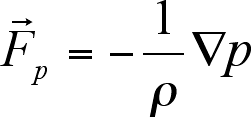with $\rho$ being the density and $p$ being the pressure. The symbol ∇ is called Nabla and is a vector differential operator. Depending on the domain in which it is applied, it denotes various quantities, for example the gradient of a scalar field, or the divergence of a vector field.

The Coriolis force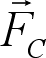can be regarded as the difference between centrifugal force and gravitation [4, p. 184]. Because of the Earth's rotation, air moving above the planet is deflected sideways, forced to follow a curved path. In the Northern hemisphere, air is deflected to the right of its direction of motion; in the Southern hemisphere, it turns to the left. The Coriolis force accelerates wind in the horizontal; it is highest at the poles and zero at the equator due to the Earth's nearly spherical shape. In mathematical terms, it is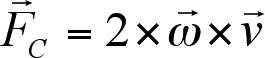with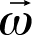being the angular velocity of a rotating system (here of the Earth) andbeing the velocity of an object relative to the system.

The air in the boundary layer, i.e. in the "air belt" of 1-2 km altitude above the Earth's surface, is exposed to drag against the ground. The drag forceincreases with wind speed, but acts against the wind direction, thereby decelerating the wind.

The termrepresents the gravitational force.

Thus, the equation of motion can be rewritten as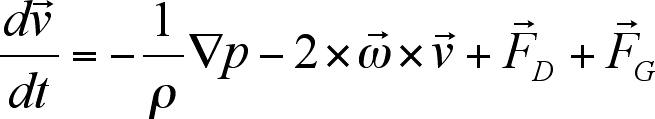Customarily, dynamical processes in the atmosphere are described in an orthogonal coordinate system rotating in time with the Earth. Therefore spherical coordinates are used.

The three components of wind velocity are written as follows: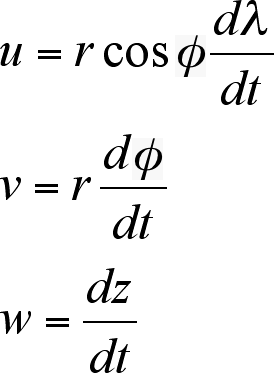Here, $u$ is the zonal wind, parallel to the circles of latitude; $v$ is the meridional wind, parallel to the circles of longitude; and $w$ is the vertical wind component . The latitude is denoted by $\phi (-\pi/2 < \phi < \pi/2)$, the longitude by $\lambda (-\pi < \lambda < \pi)$ and the height above the Earth's surface by $z$. Their changes with respect to time are indicated by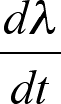,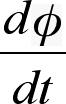andrespectively. The distance between the air parcel and the geocentre is given by $r$, where $r = R_{E} + z$ ($R_{E}$ being the Earth's radius). Often, this distance is approximated by the Earth's radius, so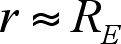. In the following formulae, I will use $r$ for better legibility.

In many forecasting models, hydrostatic equilibrium is assumed, meaning that the gravitational and the pressure gradient forces are balanced. For the vertical component of velocity $w$ we then have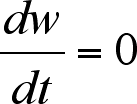.

Then the equations of motion in spherical coordinates are: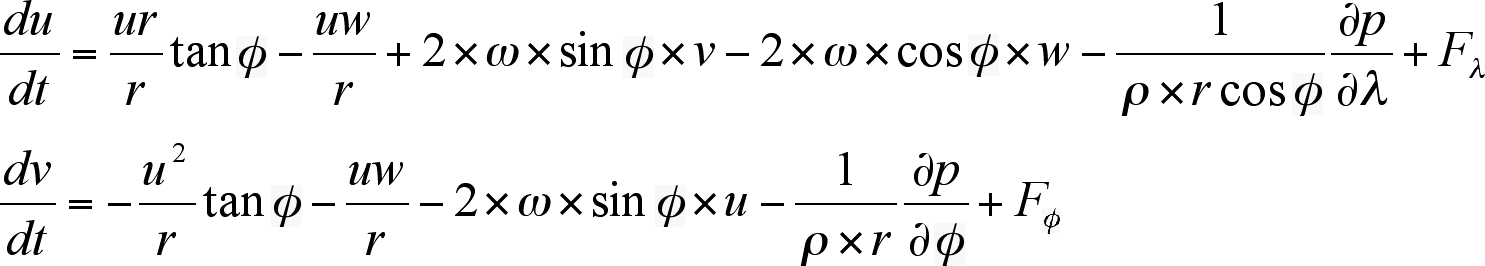where $F_{\lambda}$ and $F_{\phi}$ are components of frictional force. For predicting the motion of winds at discrete points, a so-called Eulerian reference frame is used. In this frame, the time rate of change of any quantity is expressed by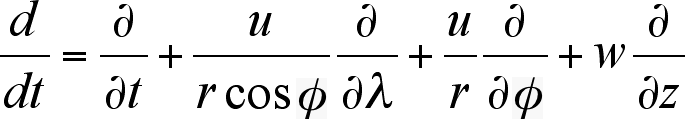The Nabla operator can also be expressed in spherical coordinates aswhere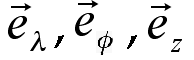are the normalised unit vectors for the directions $\lambda, \phi$ and $z$, respectively.

The basic principle underlying the continuity equation is the conservation of mass. Fundamentally, matter can be neither created out of thin air nor can it be destroyed completely; but it can be rearranged. If you want to build a house, for example, you have to use material that has already existed in some form, but through the building process it becomes a house. And if you burn a tree trunk, the wood does not vanish completely as ashes will always remain. The situation is similar for the atmosphere; if the atmospheric pressure rises in one place, it has to decrease in some other place so as to guarantee the balance. This means that if you subtract the mass flowing out of an air parcel from the mass that flew in, you obtain the change of mass within the parcel [4, p. 196]. The continuity equation is used to determine the air density.

In the Eulerian reference frame, the continuity equation is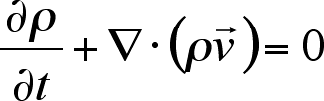This can be approximated (filtering weak acoustic waves) by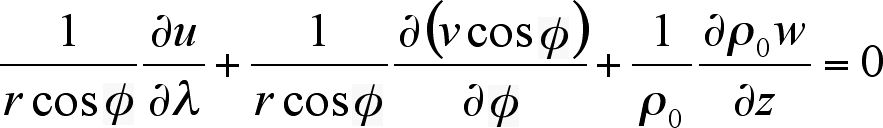where $\rho$ is the base-state density, an exponentially decreasing function of height. Substituting the continuity equation by the above filter condition is called anelastic approximation [6, p. 7].

The pressure in an air parcel is found using the equation of state, which relates pressure, temperature and density. Air behaves similar to a perfect gas, so the ideal gas law for perfect gases can be used to determine atmospheric pressure $p$: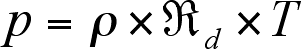Here, $T$ denotes the absolute temperature (in Kelvin); and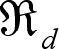is the gas constant for dry air with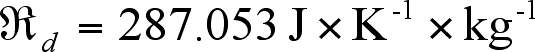. The gas constant is different for moist air, as water vapour is less dense than dry air. But instead of using a different gas constant, a virtual temperature defining this effect can be used.

In order to determine the temperature in an air parcel, forecasters make use of the first law of thermodynamics. This law expresses the conservation of energy, or heat, as heat is a form of energy. In essence, energy cannot be created or destroyed (similar to mass), but it can change forms. For example, if you have a bonfire, you need to input energy in the form of wood, and energy is released in the form of heat and light. The first law of thermodynamics also states that the amount of energy added to a system cannot be bigger than the amount of energy released from the system, in other words, the amount of energy supplied is exactly balanced by the work done (within the system).

In terms of the atmosphere, the conservation of energy means that the temperature in an air parcel changes only when heat is added or removed. This can be caused by warmer or cooler air moving into the parcel, or by evaporation (which cools the air) or condensation (which releases heat). Furthermore, the temperature in a parcel can change if the parcel moves about vertically. This property is very important for the formation of clouds [4, p. 45].

The equation describing the change of temperature $T$ with respect to time $t$ is: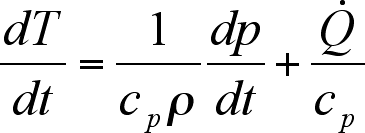Here, $c_{p}$ is the specific heat of air, with constant pressure, and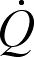is the diabatic rate of latent heat release per unit mass. The term latent heat means hidden heat, and describes the amount of energy released or absorbed by a substance -- water, in the case of the atmosphere -- during a change of state of that substance. Latent heat is released during condensation and fusion, for example; and is absorbed during evaporation and melting. "Diabatic" means that heat is transferred. The other processes in the equation are adiabatic, meaning that there is no heat transfer.

The primitive equations were first used in a weather forecast by Lewis Fry Richardson. Jule Charney and his colleagues had simplified them so that the early computers could handle them, but nowadays meteorologists have gone back to use all of Richardson's equations.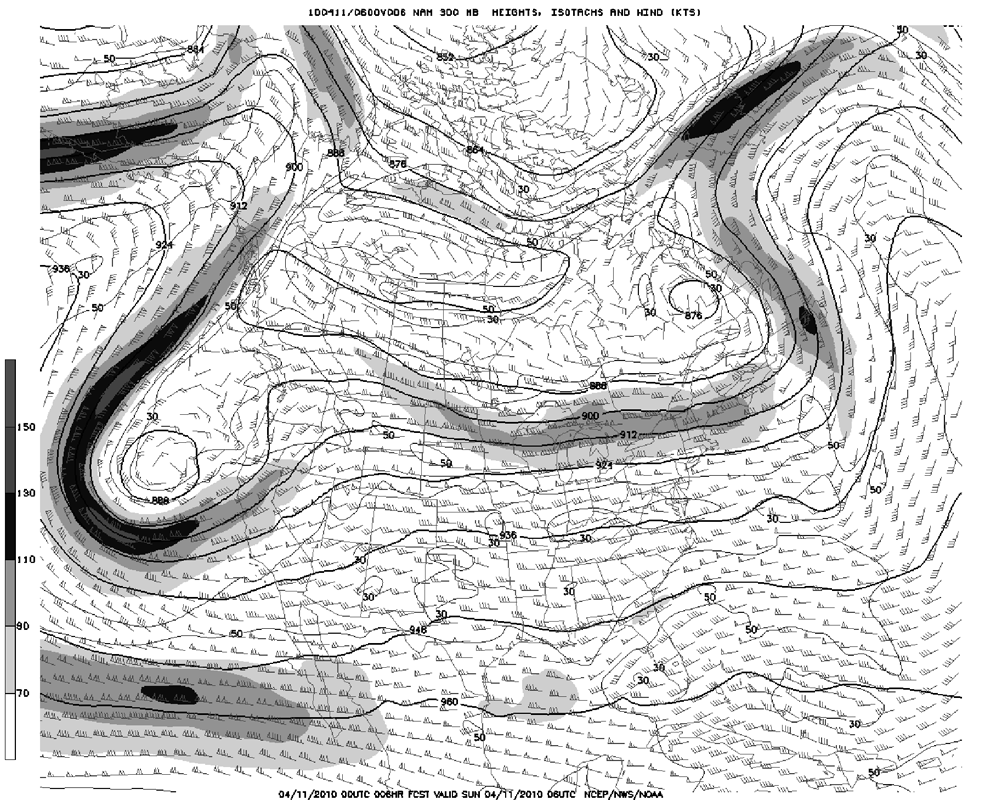A six-hour forecast for North America for 11 April 2010, with fine resolution,
produced on 11 April 2010 using the North American Mesoscale Model (NAM)
of the U. S. National Oceanic and Atmospheric Administration (NOAA).

Click on the picture to see a larger version

All current weather forecasting models are based on the primitive equations -- or versions thereof -- but each model uses different approximations and assumptions, resulting in slightly different outcomes. Also, the models include equations accounting for the effects of small-scale processes such as convection, radiation, turbulence and the effects of mountains that cannot be represented explicitly by the forecasting models, as their resolution is not high enough. This process is called parameterisation.

Furthermore, before weather data gathered from various observations can be entered into the computer models, they have to be assimilated. During data assimilation, real observations are combined with predicted conditions so as to give the best possible estimate of the actual state of the atmosphere. This process is necessary, as inputting raw data obtained just from observations results in inaccurate forecasts [4, p. 318].

Assuming that hydrostatic balance applies limits the smallest possible grid spacing to about 5 - 10 km , which is not fine enough a resolution for detailed, accurate forecasts of small-scale weather events like thunderstorms.

Some models, such as the regional model COSMO developed by the German weather service Deutscher Wetterdienst (DWD), have therefore abandoned this assumption and are based on non-hydrostatic thermodynamic equations (similar to the equations used in fluid mechanics). This permits a much finer resolution (i.e. smaller grid spacing), but as a result, the primitive equations are much more complex and computationally more demanding as vertical wind components are included in the model .
Moreover, in order to improve the model's forecast accuracy and quality, the vertical coordinate $z$ can be replaced by the generalised vertical coordinate ζ, allowing the orthogonal spherical coordinate system to be transformed into a terrain-following coordinate system, which is conformal to the Earth's orography (coordinate systems will be presented in chapter 4). Again, the primitive equations have to be re-written in terms of ζ [6, pp. 31-32].

Not all forecasting models have such a coordinate system, though; there are several grid models. But let us now look at the methods employed by meteorologists to solve these equations.

4)      Forecasting Models Used Today

For his forecasting model, Richardson constructed a grid to divide the atmosphere into many small air parcels. In essence, this is still done by meteorologists today, but several different grid versions have been developed. Similarly, Richardson's finite difference method is not the only method for solving the primitive equations anymore; its strongest "opponent" is the so-called spectral method, which will be described in section 4.2. A third method, the finite element method, will be presented briefly in section 4.3.

Before the primitive equations can be solved, they have to be discretized with respect to space and time. Discretization means that the atmosphere (or the part of it that you want to study) is represented by a finite number of numerically approximated values.

The most common numerical time integration scheme in meteorology is the leapfrog scheme. In order to make a forecast for the future (at time step $t + \Delta t$), you do not start at the present time step $t$, but at the previous step $t - \Delta t$, and the forecast leaps over the time step $t$ (with $\Delta t$ denoting the size of the time step, that is the difference between two points in time). The scheme is applied both in the finite difference method and in the spectral method.

All the different methods need to be stable, in other words, it has to be guaranteed that the numerical solution does not diverge from the true solution as the time span for which the forecast is made increases. There are various stability criteria for atmospheric processes such as advection and wave propagation. One of the main causes for instability are truncation errors, which happen when a variable ψ is represented by a Taylor series, i.e. as an infinite sum of its values at the individual grid points. Due to computational reasons, only the very first terms of the series, which are in fact the most important ones, can be used, but the higher-order terms influence the accuracy of the series. As a second order scheme, the leapfrog scheme is fairly accurate; an even better scheme is the so-called Runge-Kutta method, which is of fourth order (but it will not be described here). However in most of the current models only second order schemes can be applied due to computer capacities.
The accuracy of all forecasting models is tested regularly; statistical models have been developed for this.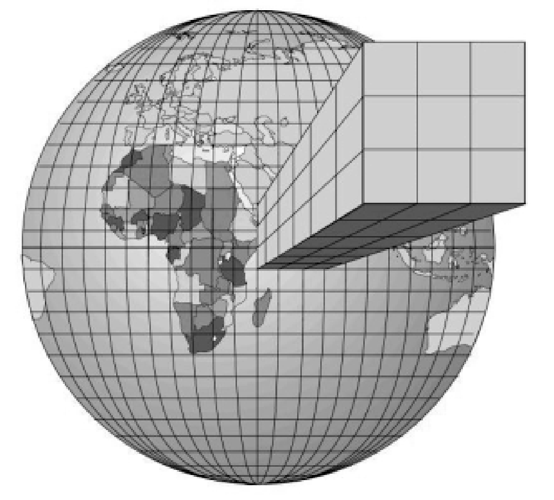A rectangular grid around the Earth with air columns above the surface.

The mathematical methods described in the subsequent sections are based on notes published by the European Centre for Medium-Range Weather Forecasts (ECMWF) in Reading, and by the German weather service DWD.

4.1)      The Finite Difference Method

The traditional grid structure is based on dividing the Earth's surface into a large number of squares, such that there is a high air column above each square, as illustrated in figure 6. The atmosphere is then divided into a number of layers, resulting in a three-dimensional grid, in which the primitive equations can be solved for each grid point. In general, the layers are much thinner close to the Earth's surface than the layers in the upper atmosphere, as most weather events happen relatively close to the ground. Processes in the upper atmosphere influence the weather, though, so the whole of the atmosphere has to be considered in a forecasting model. Most models also include several subterranean layers so as to take the air and water exchange between atmosphere and ground into account. Over the years, the resolution of the grids has become higher (i.e. the edge length of each square has become smaller).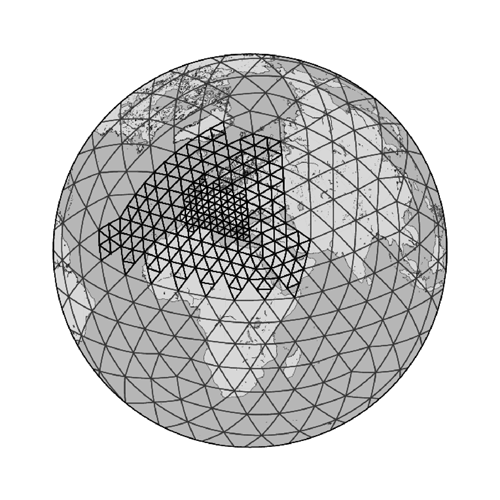A triangular grid as used by the DWD.

The world's leading weather services such as the British Met Office and the German DWD use three different grids: a global grid spanning the whole planet, a so-called regional model covering Europe (and North America in the case of the Met Office's model), and a local model covering the UK or Germany, respectively. The regional model of the DWD has a resolution of 7 km, and the local model has a resolution of up to 2.8 km (the Met Office's models have coarser resolution as the Met Office does not work with non-hydrostatic equations). Both of these local models, and all of the Met Office models, are based on a rectangular grid, whereas the DWD's global model is based on a triangular grid with a 40 km resolution (see figure 7). The great advantage of the triangular grid is that the primitive equations can be solved in air parcels close to the poles without any problems, as opposed to the rectangular grid, where the longitudes approach each other, resulting in erroneous computations.

Generally, in a terrain-following coordinate system, the grid-spacing in the λ-direction is given by Δλ; similarly, Δφ and Δζ represent the spacing in the φ-direction and the ζ-direction, respectively. As in section 3.3, λ denotes the longitude, φ denotes the latitude and ζ is the height coordinate. The position of the grid points in the computational space is defined by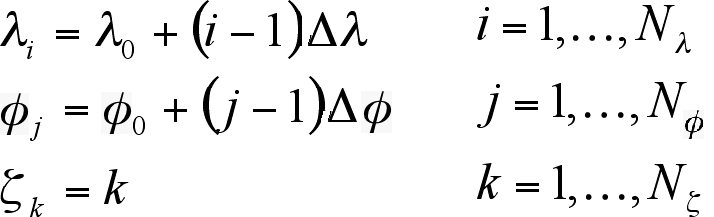Here, $N_{\alpha}$ is the number of grid points in the α-direction; λ0 and φ0 are the values of λ and φ in the southwest corner of the model domain [6, p. 53].

Now, the primitive equations have to be re-written in finite difference form. For this, we need to define approximations to the derivatives at a specified grid point $x_{l}$ in terms of finite differences. The value of a variable ψ at $x_{l}$ is given by $\psi_{l}$; and the finite difference for $\psi_{l}$ is given using the values of $\psi_{l+1}$ and $\psi_{l-1}$, i.e. the values at the two adjacent grid points. The behaviour of these two terms can be described using Taylor expansion:Subtracting the second from the first expansion gives the centred finite difference approximation to the first derivative of $\psi_{l}$: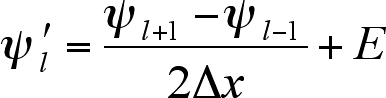The term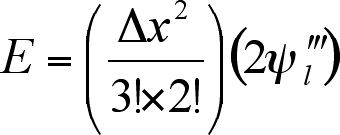gives the truncation error and can be omitted since it is small. However, the lowest power of the difference of $x$, $\Delta x$, in $E$ gives the order of the approximation. So this scheme has order 2. As mentioned above, the higher the order, the more accurate the approximation. In general, centred finite difference approximations are better than forward or backward approximations, which can also be derived from the Taylor expansions for $\psi_{l+1}$ and $\psi_{l-1}$.

Solving the first expansion for $\psi'_{l}$ gives the forward approximation: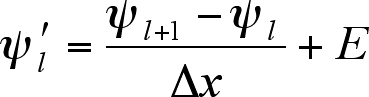Similarly, the backward approximation is derived from the second expansion: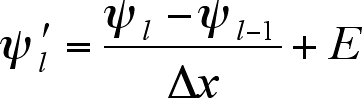These schemes both have order 1, but there are situations where it is favourable to use these approximations instead of a centred approximation. It is also possible to derive the finite difference approximations to the second and third derivatives of $\psi_{l}$.

Not only space, but also time has to be discretized, and time derivatives can also be represented as finite difference approximations, that is in terms of values at discrete time levels [17, p. 15]. A time step is denoted by $\Delta t$, and a discrete time level is given by $t_{n} = t_{0} +n\Delta t$ with $t_{0}$ being the initial time for integration. The grid point value of the variable $\psi_{l}$ at time step $t_{n}$ is denoted by $\psi^{n}_{l}$; its derivative can be expressed as a centred finite difference approximation: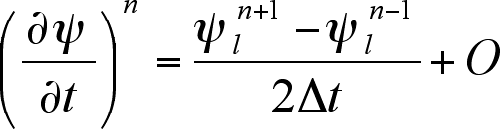Again, $O$ represents the truncation error and can be omitted.

There are two different finite difference schemes: the explicit scheme and the implicit scheme. The explicit scheme is much easier to solve than the implicit one, as it is possible to compute the new value of $\psi_{l}$ at time $n+1$ for every grid point, provided the values of $\psi_{l}$ are known for every grid point at the current time step $n$ [17, p. 15]. But the choice of the time step is limited in order to keep the scheme stable. The implicit scheme, on the other hand, is absolutely stable, but it results in a system of simultaneous equations, so is more difficult to solve [17, p. 27]. Both explicit and implicit schemes are used in current forecasting models.

The approximations described above are very simple examples illustrating the general idea of finite differences. When the primitive equations are expressed in terms of finite differences, the equations soon become very long and take some computational effort to solve. Explicit time integration can be made more efficient, though, by implementing a so-called mode-splitting technique. This means that the primitive equations are subdivided into forcing terms $f_{\psi}$ referring to slowly varying modes and source terms $s_{\psi}$ directly related to the fast-moving sound waves: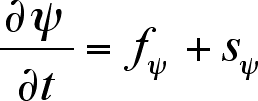. The terms $f_{\psi}$ are integrated over big time steps \Delta t. These time steps are then subdivided into several small time steps $\Delta \tau$, over which the terms $s_{\psi}$ are integrated [6, pp. 61-64].

In the case that $s_{\psi}$ = 0, we get, using a $2\Delta t$ leapfrog interval,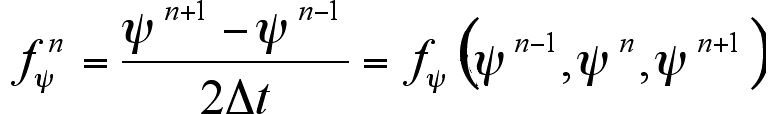representing a set of equations that can be solved using Gaussian elimination [6, p. 63].

For equations including acoustically active terms, i.e. where acoustic and gravity waves have to be taken into account, the finite difference is given byThe superscript $m$ is the time step counter for the integration over the small time steps $\Delta \tau$ within the leapfrog interval used above.

The term $f ^{n}_{\psi}$ is constant throughout the small time step integrations, but the value of $\psi^{n+1}$ is not known before the last one of these integrations has been completed. Therefore, the finite difference for $f ^{n}_{\psi}$ has to be re-written as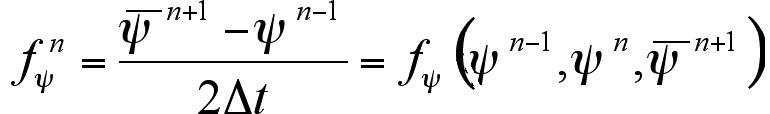The term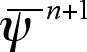is the result of a process called averaging; you assume that the mean value of $\psi^{n+1}$ does not vary as fast with respect to both space and time than deviations from the mean would. The notation for averaging iswith $a$ being an integer. The notation is similar for the longitude λ.

If you re-write the primitive equations using finite differences, you get, "after considerable algebra" [6, p. 67], a linear tridiagonal system of simultaneous equations which can be written in the general form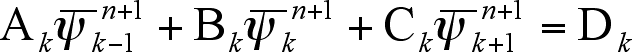The terms $A_{k}, B_{k}$ and $C_{k}$ are matrix diagonals, whereas $D_{k}$ is an inhomogeneous term including the appropriate boundary conditions. The equation system can be solved forusing a solving method based on Gaussian elimination and back-substitution.

In a nutshell, the derivatives in the primitive equations can be approximated by finite differences, such that the equations can be transformed into a linear equation system. It takes modern supercomputers at the leading weather services quite a while to solve all these equations, so it is astonishing that Richardson managed to produce a numerical weather forecast at all, even if it was for a limited area. However, the application of this method to the primitive equations was crucial to the development of numerical weather forecasting, as it was the only mathematical method that could simplify partial differential equations needed for forecasting for several decades.

A further disadvantage of the finite difference method, other than the great number of equations you have to solve, is that it does not reveal anything about the behaviour of the variables between the individual grid points. The spectral method, on the other hand, takes this into account.

4.2)      The Spectral Method

The spectral method was already devised in the 1950s, but it took a while before the method was implemented in forecasting models. In 1976, the Australian and Canadian weather services were the first ones to adopt this method, which is now used by a range of weather services across the globe; the European Forecasting Centre ECMWF in Reading, for example, adopted it in 1983 . One of the advantages of the spectral method is that the primitive equations can be solved in terms of global functions rather than in terms of approximations at specific points as in the finite difference method. For the ECMWF, this is the better option as they require a global model in order to produce medium-range weather forecasts.

For the spectral method, the atmosphere has to be represented in terms of spectral components. In the ECMWF model, the atmosphere is divided into 91 layers (in comparison, the DWD's and the Met Office's global models have 40 layers), with the number of layers in the boundary layer equalling the number of layers in the uppermost 45 km of the atmosphere. The partial differential equations are represented in terms of spherical harmonics, which are truncated at a total wave number of 799. This corresponds to a grid length of roughly 25 km  (the DWD's and the Met Office's global model has a resolution of 40 km).

In essence, in using the spectral method, you assume that an unknown variable $\psi$ can be approximated in terms of a sum of $N+1$ linearly dependent basis functions $\psi_{n}(x)$: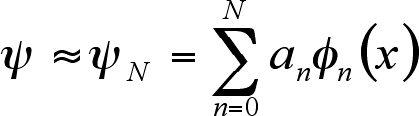When this series is substituted into an equation of the form $L\psi = f (x)$, where $L$ is a differential operator, you get a so-called residual function:
$R(x: a_{0}, a_{1}, ... , a_{N}) = L\psi_{N} - f$
The residual function is zero when the solution of the equation above is exact, therefore the series coefficients $a_{n}$ should be chosen such that the residual function is minimised, i.e. that it is as close to zero as possible. In the majority of cases, polynomial approximations, such as Fourier series or Chebyshev polynomials, are the best choice; but when it comes to weather forecasting, the use of spherical coordinates demands that spherical harmonics are used as expansion functions. This increases the complexity of the problem, and the computational effort required to solve it.

A simple example that can be solved in terms of a Fourier series illustrates the idea of the spectral method: One of the processes described by the primitive equations is advection (which is the transport of for instance heat in the atmosphere), and the non-linear advection equation is given by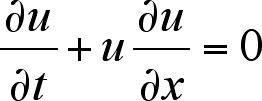This can be re-written in terms of the longitude λ: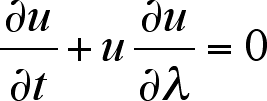Having chosen appropriate boundary conditions, the equation can be expanded in terms of a finite Fourier series: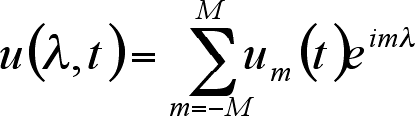where the $u_{m}$ are the complex expansion coefficients and $M$ is the maximum wave number [17, p. 61]. The advection equation then is: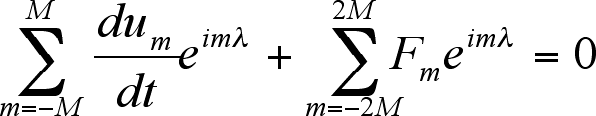where $F_{m}$ is a series in terms of the $u_{m}$.

As each of the terms on the left-hand side of the equation has been truncated at a different wave number, there will always be a residual function. There are several methods which convert differential equations to discrete problems, for example the least-square method or the Galerkin method, and which can be used in order to choose the time derivative such that the residual function is as close to zero as possible [17, p. 63].

It is difficult to calculate the non-linear terms of a differential equation in the context of the spectral method, but you can get around this problem by using a so-called transform method. Most commonly, Fast Fourier Transforms are used, but in principle all transform methods make it possible to switch between a spectral representation and a grid-point representation [17, p. 64]. Using a transform method requires three steps, which will be shown for the non-linear term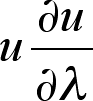in the advection equation above:

Firstly, the individual components of the non-linear term $u$ and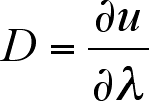are expressed in terms of spectral coefficients at discrete grid points $\lambda_{i}$: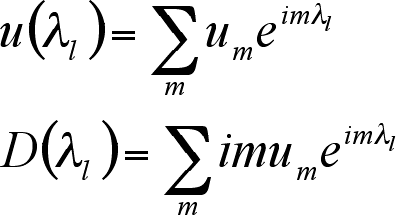Secondly, the advection term, that is the product of these components, is calculated at every grid point in the discretizised space: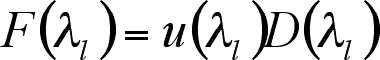Then you can return to the spectral space and calculate the Fourier coefficients: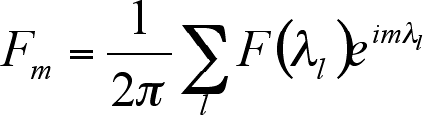This procedure has to be done at every time level, so results in a significant amount of calculation. Furthermore, products with more than two components suffer from aliasing, meaning that waves that are too short to be resolved for a certain grid resolution falsely appear as longer waves [17, p. 37]. Still, using transform methods is necessary in order to solve differential equations in spectral space.

As has been mentioned above, a dependent variable ψ has to be expanded in terms of spherical harmonics rather than Fourier coefficients when spherical coordinates are used. Spherical harmonics $Y_{n}^{m}(\lambda, \phi)$ are the angular part of the solution to Laplace's equation. The vertical components of velocity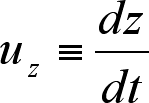transform like scalars, so can be expanded in terms of spherical harmonics straightaway. It is slightly more complicated for the horizontal components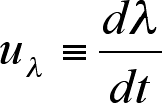and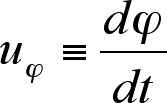: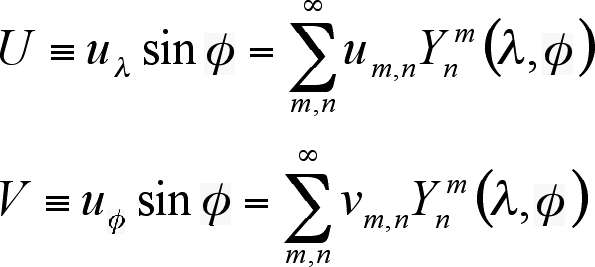[5, p. 395] with the spherical harmonics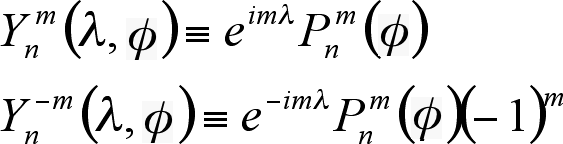where $m$ and $n$ are non-negative integers such that $n ≥ m$ [5, p. 399]. Here, $m$ is the zonal wave number, and $n$ is the total wave number. The term $P_{n}^{m}(\phi)$ is the associated Legendre function used in spherical harmonics (but will not be explained here).

Expansion in terms of spherical harmonics requires transform methods as well, but these methods are more complicated and computationally more expensive than Fast Fourier Transforms (although the use of transform methods greatly reduces the time required for calculations).

Spherical harmonics are two-dimensional, so they are much more difficult to solve than expansions in terms of Fourier series. But:
... there is no way to solve problems on the sphere that does not require some complicated programming and careful attention to detail. [5, p. 399]
In general, spectral method algorithms are more difficult to program than their finite difference counterparts; also the domains in which they are used have to be regular in order to keep the high accuracy of this method. However, the spectral method has a number of advantages: for example, there is no pole problem when the method is used. At the poles, the solutions to differential equations become infinitely differentiable; therefore the poles are usually excluded from the spectral space, which actually simplifies the method [5, p. 383]. Furthermore, it can handle finite elements of higher orders than the finite difference method can. As a result, the solutions of many problems are very accurate. The high accuracy also results in the fact that the models do not need as many grid points as in the finite difference method, and computers on which the method is run require less memory space.

Summing up, the spectral method gives much more accurate results than the finite difference method. Many weather services still use the finite difference method though because it is much easier to implement and because "the physics is so complicated ... that purely numerical errors are a low priority." [5, p. 17]

4.3)      The Finite Element Method

A third technique for finding approximate solutions to partial differential equations and hence to the primitive equations is the finite element method. It is quite similar to the spectral method in that a dependent variable ψ is defined over the whole domain in question, rather than at discrete grid points used in the finite difference method. Moreover, a finite series expansion in terms of linearly independent functions approximates the variation of ψ within a specified element (e.g. a set of grid points) [17, p. 75]. Unlike the spectral method, the basis functions are not globally, but only locally non-zero; also they are low-order polynomials rather than high-order polynomials. The domain for which the partial differential equations have to be solved is divided into a number of subdomains, and a different polynomial is used to approximate the solution for each subdomain. These approximations are then incorporated into the primitive equations. A condition for the finite element method to work, however, is that ψ is continuous between neighbouring elements. The fact that only low-order polynomials can be used is reflected in the comparatively low accuracy, but the amount of necessary calculations is much smaller than for finite differences or for spectral methods [5, p. 4]. On the other hand, there are a number of choices for the basis functions, and depending on which functions are used, the finite element method can give very accurate results when it is applied to irregular grids. Thus, the use of this method is not restricted to triangular and rectangular grids only as is the finite difference method. This is -- currently -- probably more important in engineering and fluid dynamics, where this method is most widely used. However, scientist are constantly trying to improve existing and find new mathematical methods that model atmospheric processes better than the methods in use nowadays.

4.4)      Current Research

To get to the point, most of today's research in meteorology is devoted to improving current forecasting models. This involves the use of enhanced observation and measurement techniques as well as refining the mathematical models. Several ways to improve forecast quality are already known in theory, but they cannot be implemented due to a lack of computer power. The fastest computers in civilian use are already used by the leading weather services, meaning that many weather services, especially in developing countries, have to resort to much slower computers. Since the 1960s, when issuing numerical weather forecasts calculated by computers on a regular basis begun, forecast accuracy has been accompanied by the development of faster computers, and it seems that this will be the case for the foreseeable future.

One of the easiest ways to increase the quality of weather predictions is to increase the orders of the numerical approximations to partial differential equations. Most schemes used in current models are of second order, but using third-order schemes would greatly improve forecast accuracy . In addition, both data collection and data assimilation are constantly improved. Thereby, the initial conditions entered into forecasting models represent the actual state of the atmosphere more accurately, which leads to better forecasts .

More detailed and more accurate mathematical methods as well as increased computer power will allow meteorologists to increase the resolution of their grids. A finer resolution will in turn allow them to take local weather phenomena such as thunderstorms as well as the effects caused by topographic features such as mountains and lakes into account. Ultimately, this will result in very detailed forecasts -- so meteorologists hope -- for very small specific regions. Most models include as many topographical features as possible, but according to the DWD, there is a new grid model using horizontal planes cutting through mountains, permitting a more accurate representation of the equilibrium of the atmospheric forces in the proximity of mountains. Admittedly, increasing the grid resolution involves the risk that errors in the initial data are multiplied when more grid points are used , so mathematical models will have to take this into account.

Apart from increasing the grid resolution, meteorologists also work towards increasing the time span for which forecasts can be made. The two weeks limit conjectured by Lorenz still stands; currently the achievable limit is considered to be about ten days. In February 2010, the ECMWF successfully produced accurate ten-day forecasts for the first time ever. The accuracy of a medium-range forecast is measured in terms of the anomaly correlation coefficient (ACC), which has to be above 60% in order for a forecast to be considered accurate. The February forecasts of the ECMWF were consistently above this limit .

Of course, fundamental research both in mathematics and in physics concerning atmospheric processes and the underlying mathematical and physical laws will continue to be crucial for the development of better forecasting models. A team of international researchers, led by Shaun Lovejoy of McGill University in Montreal, follows a different path than most scientist studying atmospheric processes. Interestingly enough, their research project is based on a theory by Lewis Fry Richardson. Apart from founding numerical weather forecasting, Richardson devoted a considerable amount of time to studying the atmosphere, in particular eddies and whirls. He noticed that atmospheric phenomena, for example clouds, seem to be cascade-like processes, with large-scale structures containing many ever-smaller copies of themselves, like Russian dolls sitting inside each other. In modern mathematics, this kind of phenomenon is called a fractal and its behaviour can be described using power laws (meaning that one quantity changes as another quantity is raised to some power). Richardson did not have the mathematical means to prove his assumptions, and mathematicians have only recently begun to investigate if the atmosphere can be described by a set of power laws. In a report published in 2009, Lovejoy's team provides evidence that rainfall is indeed a collection of fractals. The team's ultimate goal is to implement fractals and power laws in forecasting models in order to model small-scale processes and hence improve forecasting accuracy .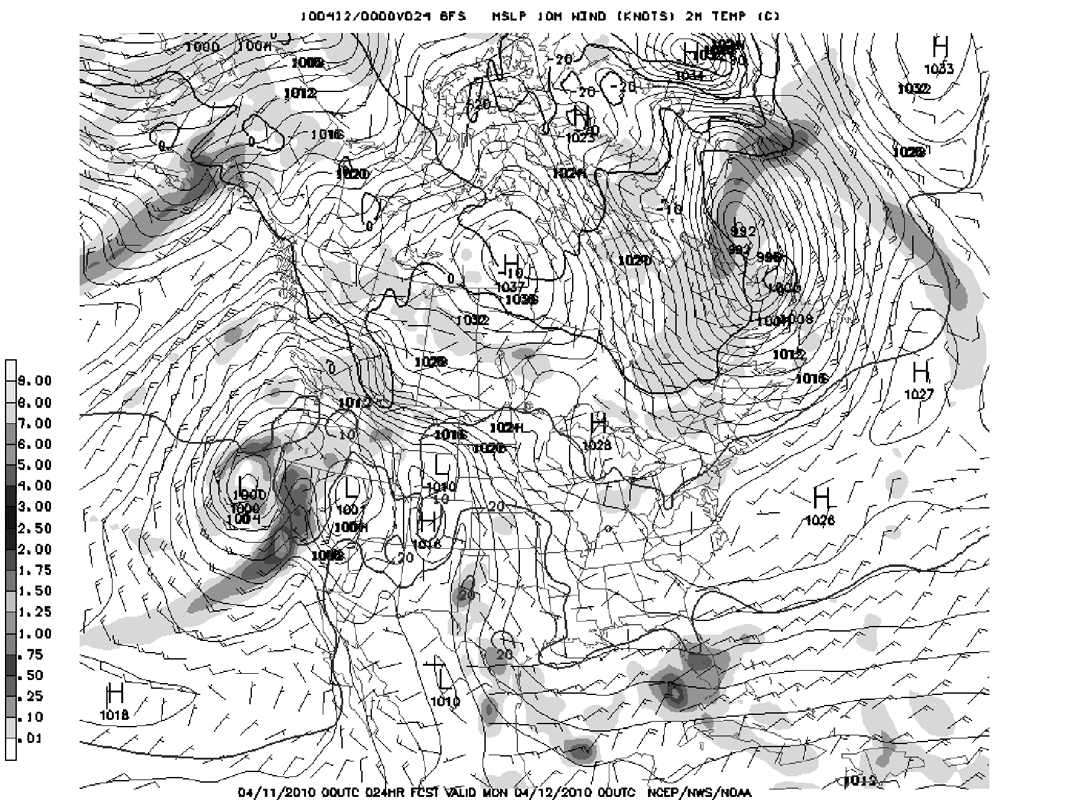The 24-hour forecast for North America for 12 April 2010 with medium resolution,
produced on 11 April 2010 using the NOAA's Global Forecast System (GFS)

Click on the picture to see a larger version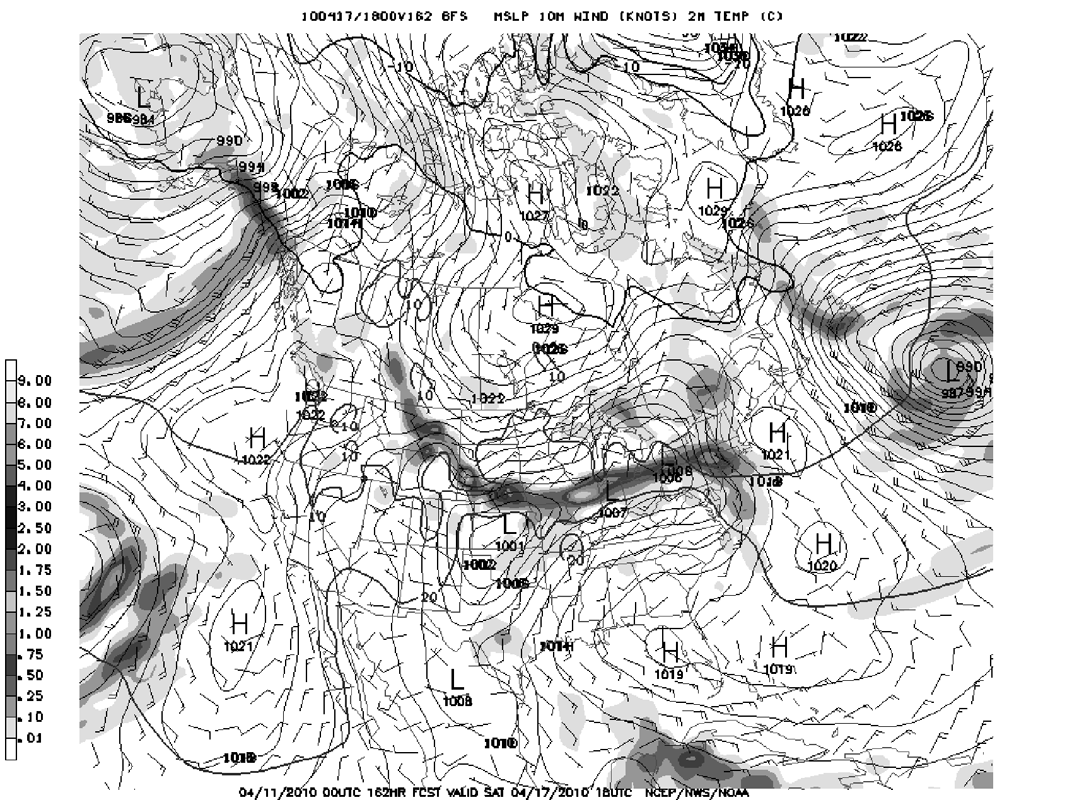A 162-hour forecast for North America for 17 April 2007 with medium resolution,
produced on 11 April 2007 using the NOAA's Global Forecast System.

Click on the picture to see a larger version

5) Conclusion

Weather forecasting has come a very long way since the Babylonians and the Greeks started observing the skies, and it was the pioneering work of Vilhelm Bjerknes and Lewis Fry Richardson at the beginning of the 20th century that kicked off the development of modern weather forecasting. But without the invention and subsequent improvement of computers, numerical weather prediction would still be in its infancy. Understanding chaos and developing new and better weather observation methods also contributed to improving forecast accuracy. A six-day forecast nowadays is now as accurate as a one-day forecast in 1968 . Current one-day forecasts are accurate in 9 out of 10 cases, and three-day forecasts still have a hit rate of 70%. Current research suggests that these percentages will continue to increase in future. Apart from normal weather forecasts, weather services also issue specialised forecasts for a variety of domains, such as agriculture, aviation and shipping, which help save lives and money. Still, meteorologists at the ECMWF estimate that the global economic loss due to inaccurate weather forecasts amounts to up to a billion Euros per year , so meteorologists hope that improved forecast quality will reduce this number.

The two different types of forecasting models, one of them based on finite differences, the other one based on the spectral method, are currently competing as to which one of them yields more accurate forecasts for a given computational cost. But at the end of the day, each model has its strengths and weaknesses; so using both models side by side will probably give the best results.
In the future, weather forecasts will be even more accurate and more detailed than forecasts nowadays. And who knows, maybe one day mathematicians will find a way to overcome the two weeks forecasting limit, so that long-range forecasts can be produced.

### References (show)

1. J D Cox, Stormwatchers. The Turbulent History of Weather Prediction from Franklin's Kite to El Niño (Hoboken NJ, 2002)
2. S Dunlop, A Dictionary of Weather, second edition (Oxford, 2008)
3. A E Gill, Atmosphere-Ocean Dynamics (San Diego CA, 1982)
4. R B Stull, Meteorology for Scientists and Engineers, second edition (Pacific Grove CA, 2000)
5. J P Boyd, Chebyshev and Fourier Spectral Methods, second edition (Mineola NY, 2000) http://www-personal.umich.edu/~jpboyd/BOOK_Spectral2000.html
6. G Doms, U Schättler, A Description of the Nonhydrostatic Regional Model LM. Part I: Dynamics and Numerics (Deutscher Wetterdienst, Offenbach, 2002) http://www.dwd.de/bvbw/appmanager/bvbw/dwdwwwDesktop?_nfpb=true&_pageLabel=_dwdwww_aufgabenspektrum_forschung&T22800286021158037121504gsbDocumentPath=Content%2FForschung%2FFE1%2FVeroeffentlichungen%2FDownload%2FLMdocu__I__dynamics__0211.html
7. F Grazzini, A Persson, User Guide to ECMWF forecast products, version 4.0 (Meteorological Bulletin M3.2, 14.03.2007) http://www.ecmwf.int/products/forecasts/guide/user_guide.pdf
8. J C R Hunt, Lewis Fry Richardson and his Contributions to Mathematics, Meteorology, and Models of Conflict (Annual Review for Fluid Mechanics, Volume 30, January 1998), xiii-xxxvi http://arjournals.annualreviews.org
9. C E Leith, Objective Methods for Weather Prediction (Annual Review for Fluid Mechanics, 10/1978), 107-128 http://arjournals.annualreviews.org
10. R Stewart, Weather Forecasting by Computer (2009) http://www.robinstewart.com/personal/learn/wfbc/index.html
11. A J Thorpe, H Volkert, M ZiemiaDski, The Bjerknes' Circulation Theorem: A Historical Perspective (Bulletin of the American Meteorological Society, Volume 84, Issue 4, April 2003), 471-480 http://ams.allenpress.com/archive/1520-0477/84/4/pdf/i1520-0477-84-4-471.pdf
12. M Birke, Wettervorhersage & Wetterdienst (University of Regensburg, 2009) http://www.physik.uni-regensburg.de/forschung/wegscheider/gebhardt_files/skripten/Wettervorhersage.Birke.pdf
13. B Früh, Wie lässt sich das Klima der Zukunft berechnen? (University of Karlsruhe, 2008) http://www.physik.uni-karlsruhe.de/Aktuelles/Vergangene_Veranstaltungen/Lehrerfortbildung/Lehrer_2008/LFB08_Frueh.pdf
14. R S Harwood, Atmospheric Dynamics (Chapter 1: Basics, Chapter 5: Balance of Forces in Synoptic Scale Flow, Chapter 13: Quasi-Geostrophic Equations) (University of Edinburgh, 2005) http://xweb.geos.ed.ac.uk/~rharwood/teaching/phys4/dynamics/index.html
15. N Kämpfer, Atmosphärenphysik. Kapitel 6: Dynamik der Atmosphäre (University of Bern, 2009), 118-123 http://www.iapmw.unibe.ch/teaching/vorlesungen/atmosphaerenphysik/FS_2009/
16. U Langematz, Vorlesung 6: Dynamik I (FU Berlin, 2009) www.geo.fu-berlin.de/met/ag/strat/lehre/sose09/Vorlesung_Mittlere_Atmosphaere/ MSc_Meteorologie_der_MA_V6.pdf
17. R W Riddaway, Numerical Methods, revised March 2001 (by M Hortal) (Meteorological Training Course Lecture Series, 2002) http://www.ecmwf.int/newsevents/training/course_notes/pdf_files/Numerical_methods.pdf
18. J Wensch, Numerische Wettervorhersage (TU Dresden, 2009 www.math.tu-dresden.de/~wensch/semi09W/vortrag.pdf
19. S Auyang, Nonlinear Dynamics: How Science Comprehends Chaos (talk given at Harvard University, 23.02.1998)http://www.creatingtechnology.org/papers/chaos.htm
20. F Baer, The Spectral Method: Its Impact on NWP (2004) http://www.ncep.noaa.gov/nwp50/Presentations/Tue_06_15_04/session_3/Baer_JNWP50_baer_/3.ppt
21. P Bethge, J Blech, M Dworschak, O Stampf, Das gefühlte Wetter (Spiegel, 23/2000) http://www.spiegel.de/spiegel/print/d-16597522.html
22. C Budd, Finding order in chaos http://plus.maths.org/issue26/features/budd/index.html
23. C Budd, How maths can make you rich and famous: Part II http://plus.maths.org/issue25/features/budd/
24. A Gassmann, Numerische Wettervorhersage (Welt der Physik/MPI für Meteorologie, 2008) http://www.weltderphysik.de/de/6280.php
25. R Matthews, And now, the forecast ... cloudy with a chance of fractals (NewScientist, No 2733, 7 November 2009), 40-43
26. W Wiedlich, Eine kleine Geschichte der Wettervorhersage (General-Anzeiger, 27.11.2007) http://www.general-anzeiger-bonn.de/index.php?k=wett&itemid=10592&detailed=384284
27. http://www.britannica.com/EBchecked/topic/67368/Vilhelm-Bjerknes
28. http://www.dwd.de
29. http://earthobservatory.nasa.gov/Features/Bjerknes/
30. http://earthobservatory.nasa.gov/Features/WxForecasting/wx.php, M Chahine, S Graham, C Parkinson
31. http://ecmwf.int/
32. http://encyclopedia.stateuniversity.com/pages/14958/meteorology.html
33. http://www.fi.edu/weather/owc.html
34. http://www-history.mcs.st-andrews.ac.uk/Biographies/Bjerknes_Vilhelm.html, J J O'Connor, E F Robertson
35. http://www-history.mcs.st-andrews.ac.uk/Biographies/Lorenz_Edward.html, J J O'Connor, E F Robertson
36. http://www-history.mcs.st-andrews.ac.uk/Biographies/Richardson.html, J J O'Connor, E F Robertson
37. http://www.infoplease.com/cig/weather/forecasting-from-beginning.html, M Goldstein [extract from The Complete Idiot's Guide to Weather, M Goldstein, 2002]
38. http://met.no
39. http://www.metoffice.gov.uk
40. http://www.nap.edu/html/biomems/jcharney.html, N A Philips